# (Solved Homework): M H tree reachesBoth ("B", H") would return "G tree. reach ("F", N") would return "M tree. re D") would return "D tree. reachesBoth("X", "M") would return nu

M H tree reachesBoth (“B”, H”) would return “G tree. reach (“F”, N”) would return “M tree. re D”) would return “D tree. reachesBoth(“X”, “M”) would return nu

(4 bookmarks)

1. Implement public T reachesBoth(T a, T b) You should return the data value of the node that can reach both a and b in the least number of steps (a node can reach itself in 0 steps). If a or b is not in the tree, return null. Consider the BinaryTree tree on the left with outputs on the right: tree.reachesBoth(“B”, “H”) would return “G” tree.reachesBoth(“F”, “N”) would return “M” tree.reachesBoth(“B”, “D”) would return “D” tree.reachesBoth(“X”, “M”) would return null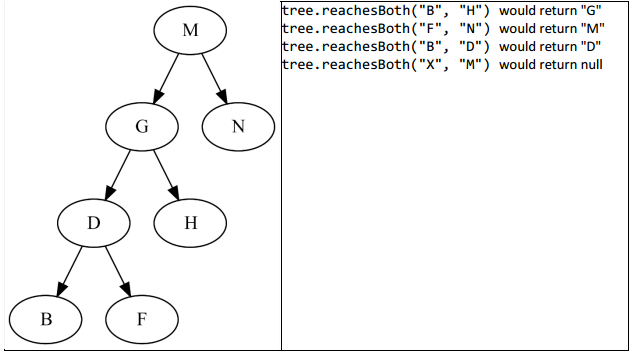2. Implement public T findRightmostLowest() In the above tree, there are two lowest nodes: B and F. Both of them are distance 3 from the root; all other nodes are less than distance 3. F is to the right of B so tree.findRightmostLowest() should return “F”.

3. Implement public T findKthLargest(int k) Consider the sorted order of all the elements in the tree. The index of the smallest element is 0. The index of the largest element is tree.size() – 1. In the above tree, tree.findKthLargest(1) would return “D” and tree.findKthLargest(4) would return “H”. Return null if k is out of range.

4.: Implement public void balance() Use a findKthLargest-based approach to rebalance the tree using a pivot-style approach. The new root should be the tree’s median (KthLargest index of size() / 2). Recursively, the root of each branch should be the median of each branch (index within that subtree of its size / 2). This method should not need to call new and should execute in O(n log n) time to receive full credit.

Thanks in advance! Will rate if good. Plz start with the following code that has not been implemented yet.

import java.util.Arrays;

/**
* A binary search tree for Comparable objects such as Strings, Integers, etc.
* For each node n, all nodes to the left have data which is less than n.data
* and all nodes to the right have data which is greater than n.data.
*
* @param
*/
public class BinaryTree> {
private static class Node> {
public T data;
public Node left, right;

int comp = d.compareTo(data);
if (comp == 0)
if (comp < 0) {
if (left == null) {
left = new Node<>();
left.data = d;
} else {
}
} else {
// Greater than
if (right == null) {
right = new Node<>();
right.data = d;
} else {
}
}
}

public boolean contains(T d) {
int comp = d.compareTo(data);
if (comp == 0)
return true; // Already in tree
if (comp < 0) {
if (left == null) {
return false; // Not in the tree
} else {
return left.contains(d);
}
} else {
if (right == null) {
return false; // Not in the tree
} else {
return right.contains(d);
}
}

}

public void print(int indent) {
if (right != null)
right.print(indent + 1);
char[] spaces = new char[indent * 2];
Arrays.fill(spaces, ‘ ‘);
System.out.println(new String(spaces) + data);
if (left != null)
left.print(indent + 1);
}

/**
* The number of nodes of this subtree.
* @return Number of nodes
*/
public int size() {
// We know there is a node here
int total = 1;
// This node may have left children
if (left != null)
total = total + left.size();
// This node may have right children
if (right != null)
total = total + right.size();
// The total size of the tree from this point…
}

/**
* Delete this node.
*
* @return The new root of this subtree (null if this node had no
* children, also known as a leaf)
*/
public Node deleteNode() {
if (left == null)
return right;
if (right == null)
return left;
Node successor = right;
if (successor.left == null) {
// Case 1: no left child of immediate successor
right = right.right;
} else {
// Case 2: loop until we find leftmost child
Node successorParent = null;
while (successor.left != null) {
successorParent = successor;
successor = successor.left;
}
successorParent.left = successor.right;
}
// Replace this data with successor data
data = successor.data;
return this;
}

/**
* Deletes the node containing d if it exists.
*
* @param d
* @return A valid BinaryTree that doesn’t have d in it but does have
* everything else.
*/
public Node delete(T d) {
int comp = d.compareTo(data);
if (comp == 0)
return deleteNode();
if (comp < 0) {
// If d exists, it’s to the left
if (left != null)
left = left.delete(d);
return this;
} else {
if (right != null)
right = right.delete(d);
return this;
}
}
}

private Node root;

public BinaryTree() {
root = null;
}

/**
* Adds data to the tree if it didn’t already contain it.
*
* @param data
*/
if (root == null) {
root = new Node<>();
root.data = data;
} else {
}
}

/**
* Returns true if the tree contains data, false otherwise
*
* @param data
* Does the tree contain this?
* @return true if it does
*/
public boolean contains(T data) {
if (root == null)
return false;
return root.contains(data);
}

/**
* Prints out a representation of the tree (rotate your head 90 degrees
* left)
*/
public void print() {
if (root != null)
root.print(0);
}

/**
* Gets the number of nodes of the tree in O(n) time.
*
* @return number of nodes
*/
public int size() {
if (root == null)
return 0;
return root.size();
}

/**
* Delete the node containing data from the tree, if it exists.
*
* @param data
*/
public void delete(T data) {
root = root.delete(data);
}

/**
* Returns the data value of the node that can reach both a and b in the
* least number of steps. If the tree doesn’t contain both a and b, return
* null.
*
* @param a
* @param b
* @return data value
*/
public T reachesBoth(T a, T b) {
// TODO: Implement.
return null;
}

/**
* Among all the nodes which are farthest from the root, find the one which
* is farthest to the right.
*
* @return data value of said node
*/
public T findRightmostLowest() {
// TODO: Implement.
return null;
}

/**
* Return the kth largest element according to the Comparable sorted order
* of the tree. The leftmost node has index 0 and the rightmost node has
* index size() – 1.
*
* @param k
* index
* @return element, or null if k is out of range.
*/
public T findKthLargest(int k) {
// TODO: Implement.
return null;
}

/**
* EXTRA CREDIT: Balance the tree. The new root should be the
* findKthLargest(size()/2) node. Recursively, the root of each subtree
* should also be the size/2-largest node (indexed from 0) of that subtree.
* This method should not call new and should execute in O(n log n) time for
* full credit.
*/
public void balance() {
// TODO: Implement for extra credit.
}
}

import java.util.Arrays;
/**
* A binary search tree for Comparable objects such as Strings, Integers, etc.
* For each node n, all nodes to the left have data which is less than n.data
* and all nodes to the right have data which is greater than n.data.
*
* @param
*/
public class BinaryTree<T extends Comparable<T>> {
private static class Node<T extends Comparable<T>> {
public T data;
public Node<T> left, right;
int comp = d.compareTo(data);
if (comp == 0)
if (comp < 0) {
if (left == null) {
left = new Node<>();
left.data = d;
} else {
}
} else {
// Greater than
if (right == null) {
right = new Node<>();
right.data = d;
} else {
}
}
}
public boolean contains(T d) {
int comp = d.compareTo(data);
if (comp == 0)
return true; // Already in tree
if (comp < 0) {
if (left == null) {
return false; // Not in the tree
} else {
return left.contains(d);
}
} else {
if (right == null) {
return false; // Not in the tree
} else {
return right.contains(d);
}
}
}
public void print(int indent) {
if (right != null)
right.print(indent + 1);
char[] spaces = new char[indent * 2];
Arrays.fill(spaces, ‘ ‘);
System.out.println(new String(spaces) + data);
if (left != null)
left.print(indent + 1);
}
/**
* The number of nodes of this subtree.
* @return Number of nodes
*/
public int size() {
// We know there is a node here
int total = 1;
// This node may have left children
if (left != null)
total = total + left.size();
// This node may have right children
if (right != null)
total = total + right.size();
// The total size of the tree from this point…
}
/**
* Delete this node.
*
* @return The new root of this subtree (null if this node had no
* children, also known as a leaf)
*/
public Node deleteNode() {
if (left == null)
return right;
if (right == null)
return left;
Node successor = right;
if (successor.left == null) {
// Case 1: no left child of immediate successor
right = right.right;
} else {
// Case 2: loop until we find leftmost child
Node successorParent = null;
while (successor.left != null) {
successorParent = successor;
successor = successor.left;
}
successorParent.left = successor.right;
}
// Replace this data with successor data
data = (T)successor.data;
return this;
}
/**
* Deletes the node containing d if it exists.
*
* @param d
* @return A valid BinaryTree that doesn’t have d in it but does have
* everything else.
*/
public Node delete(T d) {
int comp = d.compareTo(data);
if (comp == 0)
return deleteNode();
if (comp < 0) {
// If d exists, it’s to the left
if (left != null)
left = left.delete(d);
return this;
} else {
if (right != null)
right = right.delete(d);
return this;
}
}
}
private Node root;
public BinaryTree() {
root = null;
}
/**
* Adds data to the tree if it didn’t already contain it.
*
* @param data
*/
if (root == null) {
root = new Node<>();
root.data = data;
} else {
}
}
/**
* Returns true if the tree contains data, false otherwise
*
* @param data
* Does the tree contain this?
* @return true if it does
*/
public boolean contains(T data) {
if (root == null)
return false;
return root.contains(data);
}
/**
* Prints out a representation of the tree (rotate your head 90 degrees
* left)
*/
public void print() {
if (root != null)
root.print(0);
}
/**
* Gets the number of nodes of the tree in O(n) time.
*
* @return number of nodes
*/
public int size() {
if (root == null)
return 0;
return root.size();
}
/**
* Delete the node containing data from the tree, if it exists.
*
* @param data
*/
public void delete(T data) {
root = root.delete(data);
}

/*
This is a recursive helper function to compute reaches both
*/
private T reachesBothAtNode(T a, T b, Node<T> n) {
if(n==null) {
// reached at the bottom and neither a nor b were seen
return null;
}
if(a.compareTo(n.data) == 0) {
// the current node has value a
// if the node contains b anywhere then this is where it reaches both a and b
return n.contains(b) ? a : null;
}
if(b.compareTo(n.data) == 0) {
// the current node has value b
// if the node contains a anywhere then this is where it reaches both a and b
return n.contains(a) ? b : null;
}
if(a.compareTo(n.data) < 0 && b.compareTo(n.data) < 0) {
// both a and b are less than current node value
// if there’s a node that reaches both, it must be in the left subtree
return reachesBothAtNode(a, b, n.left);
}
if(a.compareTo(n.data) > 0 && b.compareTo(n.data) > 0) {
// both a and b are greater than current node value
// if there’s a node that reaches both, it must be in the right subtree
return reachesBothAtNode(a, b, n.right);
}
if(a.compareTo(n.data) < 0 && b.compareTo(n.data) > 0) {
// a is less and b is greater than current node value
// a ‘could’ be in the left and b in right subtree
// if so then our current node reaches both
return (n.left).contains(a) && (n.right).contains(b) ? n.data : null;
}
if(a.compareTo(n.data) > 0 && b.compareTo(n.data) < 0) {
return (n.left).contains(b) && (n.right).contains(a) ? n.data : null;
}
return null;
}

/**
* Returns the data value of the node that can reach both a and b in the
* least number of steps. If the tree doesn’t contain both a and b, return
* null.
*
* @param a
* @param b
* @return data value
*/
public T reachesBoth(T a, T b) {
return reachesBothAtNode(a,b,(Node<T>)root);
}

/*
A class to save right most node with its height
height will be required for comparison
*/
private static class RMLWithHeight<T> {
int height;
T data;
public RMLWithHeight(int h,T d) {
height = h;
data = d;
}
}

// recursive helper method for findRightmostLowest of the tree
private RMLWithHeight<T> findRightmostLowestAtNode(Node<T> n) {
if(n.left==null && n.right==null) {
// leaf node height is 0
return new RMLWithHeight(0,n.data);
}
if(n.left == null) {
// no node on left and hence the lowest will always be on the right
RMLWithHeight<T> rmlAtRight = findRightmostLowestAtNode(n.right);
return new RMLWithHeight(rmlAtRight.height+1,rmlAtRight.data);
}
if(n.right == null) {
// no node on right and hence the lowest will always be on the right
RMLWithHeight<T> rmlAtLeft = findRightmostLowestAtNode(n.left);
return new RMLWithHeight(rmlAtLeft.height+1,rmlAtLeft.data);
}
RMLWithHeight<T> rmlAtLeft = findRightmostLowestAtNode(n.left);
RMLWithHeight<T> rmlAtRight = findRightmostLowestAtNode(n.right);
// compare heights of the trees at left and right
// return right most node at left if has a greater height
// also update the height, its one level higher than n.left
if(rmlAtLeft.height > rmlAtRight.height) {
return new RMLWithHeight(rmlAtLeft.height+1,rmlAtLeft.data);
}
// in case right has less or equivalent height, return right most node at right
// also update the height, its one level higher than n.right
return new RMLWithHeight(rmlAtRight.height+1,rmlAtRight.data);
}

/**
* Among all the nodes which are farthest from the root, find the one which
* is farthest to the right.
*
* @return data value of said node
*/
public T findRightmostLowest() {
RMLWithHeight<T> rml = findRightmostLowestAtNode((Node<T>)root);
return rml.data;
}

public Node<T> findKthLargestAtNode(int k, Node<T> n) {
if(n==null) {
return null;
}
int i = 0; // number of nodes in left sub-tree
if(n.left != null) {
i = (n.left).size();
}

// i is the number of nodes in the tree smaller that the node n
if(i > k) {
// kth smallest element must be less that the current node and hence in the left subtree
return findKthLargestAtNode(k, n.left);
}
if(i == k) {
// kth smallest element is the current node
// since the number of elements less than the current node is k
return n;
}
if(i < k) {
// kth smallest element must be more that the current node
// and hence in the right subtree

// the index of current node is i
// and everything on right is greater that the current node
return findKthLargestAtNode(k-i-1, n.right);
}
return null;
}
/**
* Return the kth largest element according to the Comparable sorted order
* of the tree. The leftmost node has index 0 and the rightmost node has
* index size() – 1.
*
* @param k
* index
* @return element, or null if k is out of range.
*/
public T findKthLargest(int k) {
Node<T> knn = findKthLargestAtNode(k,(Node<T>)root);
if (knn == null) {
return null;
}
return knn.data;
}

public void balanceAtNode(Node<T> n, Node<T> parent, boolean isLeft) {
if(n==null) {
return;
}
int k = n.size()/2;

Node<T> mid = findKthLargestAtNode(k,n);

int i=0;
if(mid.left != null) {
i = (mid.left).size();
}

if (mid == n) {
balanceAtNode(mid.left, mid, true);
balanceAtNode(mid.right, mid, false);
} else {
Node

tmp = n;
Node prev = null;
while(true) {
if(mid.data.compareTo((T)tmp.data)<0) {
prev = tmp;
tmp = prev.left;
} else if (mid.data.compareTo((T)tmp.data)>0) {
prev = tmp;
tmp = prev.right;
} else {
break;
}
}
boolean copy_left = true;
if(i==k) {
copy_left = false;
}
if(prev.right == mid) {
if(copy_left) {
prev.right = mid.left;
mid.left = n;
} else {
prev.right = mid.right;
mid.right = n;
}
} else {
if(copy_left) {
prev.left = mid.left;
mid.left = n;
} else {
prev.left = mid.right;
mid.right = n;
}
}

balanceAtNode(mid.left, mid, true);
balanceAtNode(mid.right, mid, false);
}
if(parent == null) {
root = mid;
} else if(isLeft) {
parent.left = mid;
} else {
parent.right = mid;
}
}
/**
* EXTRA CREDIT: Balance the tree. The new root should be the
* findKthLargest(size()/2) node. Recursively, the root of each subtree
* should also be the size/2-largest node (indexed from 0) of that subtree.
* This method should not call new and should execute in O(n log n) time for
* full credit.
*/
public void balance() {
balanceAtNode((Node<T>) root, null, true);
}
}

A sample test program: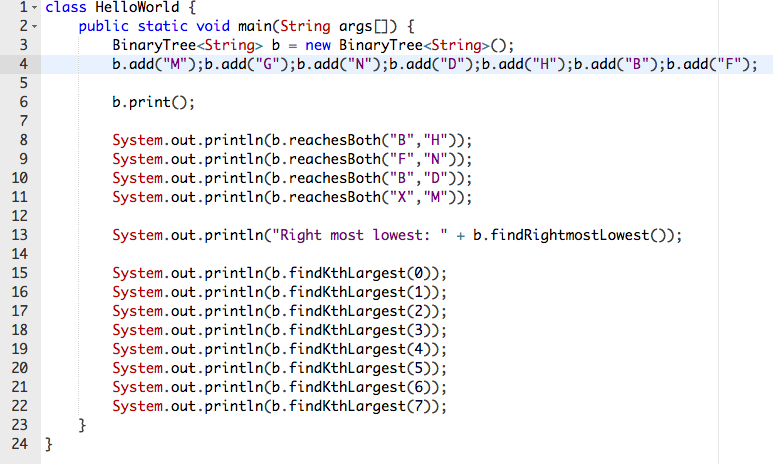Ouput screenshot: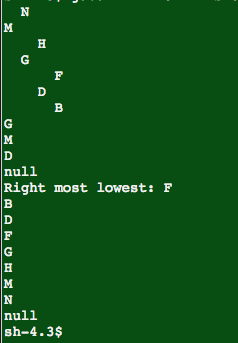sample test program to test the bonus function: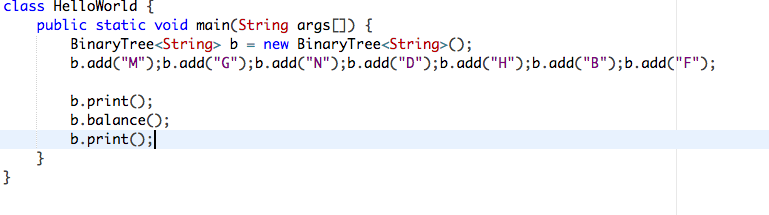Output screenshot: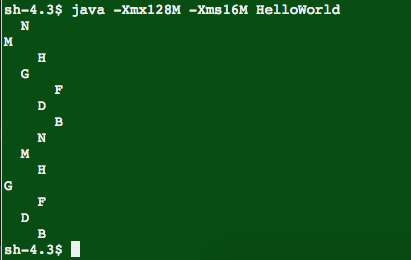Order NOW for a 10% Discount
Pages (550 words)
Approximate price: -

Why Us?Top Quality and Well-Researched Papers

All ourbpapers are written from scratch. In fact, Clients who ask for paraphrasing services are highly discouraged. We have writers ready to craft any paper from scratch and deliver quality ahead of time.Our writers keeps you posted on your papers progress - providing you with paper outline/draft. You are also at liberty to communicate directly with your writer.Free Unlimited Revisions

If you think we missed something, send your order for a free revision. You have 10 days to submit the order for review after you have received the final document. You can do this yourself after logging into your personal account or by contacting our support.Prompt Delivery and 100% Money-Back-Guarantee

All papers are always delivered on time, in many cases quite ahead of time. In case we need more time to master your paper, we may contact you regarding the deadline extension. In case you cannot provide us with more time, a 100% refund is guaranteed.Original & Confidential

We use several writing tools checks to ensure that all documents you receive are free from plagiarism. Our editors carefully review all quotations in the text. We also promise maximum confidentiality in all of our services.Our support agents are available 24 hours a day 7 days a week and committed to providing you with the best customer experience. Get in touch whenever you need any assistance.

Try it now!

## Calculate the price of your order

Total price:
\$0.00

How it works?Fill in the order form and provide all details of your assignment.Proceed with the payment

Choose the payment system that suits you most.Our Services

No need to work on your paper at night. Sleep tight, we will cover your back. We offer all kinds of writing services.## Essay Writing Service

No matter what kind of academic paper you need and how urgent you need it, you are welcome to choose your academic level and the type of your paper at an affordable price. We take care of all your paper needs and give a 24/7 customer care support system.An admission essay is an essay or other written statement by a candidate, often a potential student enrolling in a college, university, or graduate school. You can be rest assurred that through our service we will write the best admission essay for you.Editing Support

Our academic writers and editors make the necessary changes to your paper so that it is polished. We also format your document by correctly quoting the sources and creating reference lists in the formats APA, Harvard, MLA, Chicago / Turabian.Revision Support

If you think your paper could be improved, you can request a review. In this case, your paper will be checked by the writer or assigned to an editor. You can use this option as many times as you see fit. This is free because we want you to be completely satisfied with the service offered.